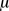SEARCH HOMEMath Central Quandaries & QueriesHi, Can anyone help me with this question. The amount of time required for a certain type of automobile transmission repair at a service garage is normally distributed with the mean = 45 minutes and the standard deviation =8.0 minutes. The service manager plans to have work begin on the transmission of a customer's car 10 minutes after the car is dropped off, and he tells the customer that the car will be ready within one hour total time. What is the probability that he will be wrong? Illustrate the proportion of area under the normal curve which is relevant in this case. What is the required working time allotment such that there is a 75 percent chance that the transmission repair will be completed within that time? Illustrate the proportion of area that is relevant. Thank you, I really appreciate it. ErikaHi Erika,

You have a normal distribution with= 45 and= 8.0. Let X be the amount of time it takes to complete the repair on a customer's car. To finish in one hour you must have X ≤ 50 so the first question is to find

Pr(X > 50).

Because of the way that the normal table is presented is is easiest to calculate this by

Pr(X > 50) = 1 - Pr(X ≤ 50).

You need to change this to a question about the standard normal variable Z using the transformation

Z = (X -)/= (X - 45)/8.0

Thus the first question can be answered by using the normal table to find

Pr(X ≤ 50) = Pr(Z ≤ (50 - 45)/8.0) = Pr(Z ≤ 0.625)

For the second question you want to find the time T so that

Pr(X ≤ T) = 0.75

Follow my steps above to convert this to a probability statement about Z carrying T in place of 50. At this point you need to use the normal table "backwards". That is you need to find 0.75, or something close to that, in the body of the table. Read the Z value from the table and solve for T.

I hope this helps,
PennyMath Central is supported by the University of Regina and The Pacific Institute for the Mathematical Sciences.